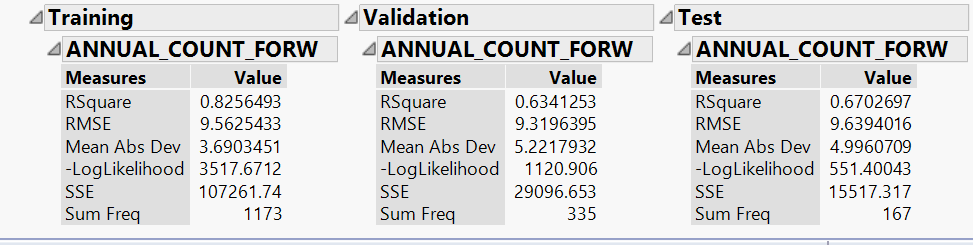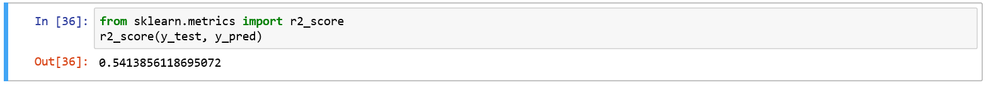Choose Language Hide Translation Bar

## Predicted R-square calculator

The predicted residual error sum of squares (PRESS) is a type of cross-validation used to evaluate the predictive ability of a regression model. Predicted r-square is a related goodness-of-fit measure. This add-in will calculate the predicted r-square from the PRESS and the total sum of squares (SST) and place it in a column next to PRESS in the report window when using Fit Model. Please note, Analysis of Variance and Press must be turned on in the report window prior to running this add-in. If needed, turn them on by selecting Regression Report > Analysis of Variance and Row Diagnostics > Press from the red triangle menu next to the response.

I can see that the Predicted R-square is already available out of the box in JMP 14 in Row Diagnostics>Press. But I really find this metric useful for comparing models, not just for one model.

Is there any way of getting "Press RSquare" to appear also in Stepwise Fit > All Possible Models, as an additional column?

Thanks!

I built a neural network model with JMP. It outputs an R square value. It outputs an R square value，like this:but when I output predicted value and test the R square in Python, I found it's wrong,like this:this is validation dataset R square I calculated using  SKlearn.

0.63 and 0.54,it's big difference.

Any staff can answer my question？

Article Labels
Article Tags
Contributors